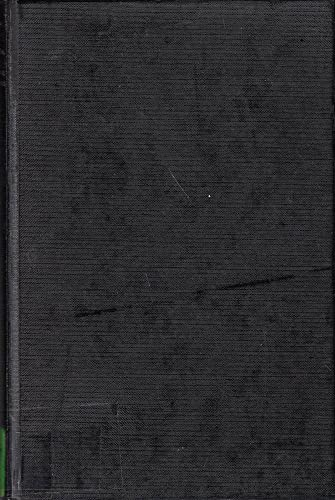# A Handbook of Numerical and Statistical Techniques: With Examples Mainly from the Life Sciences

## Pollard, J. H.

Valutazione media 0
( su 0 valutazioni fornite da Goodreads )Vedi tutte le copie di questo ISBN:

This handbook is designed for experimental scientists, particularly those in the life sciences. It is for the non-specialist, and although it assumes only a little knowledge of statistics and mathematics, those with a deeper understanding will also find it useful. The book is directed at the scientist who wishes to solve his numerical and statistical problems on a programmable calculator, mini-computer or interactive terminal. The volume is also useful for the user of full-scale computer systems in that it describes how the large computer solves numerical and statistical problems. The book is divided into three parts. Part I deals with numerical techniques and Part II with statistical techniques. Part III is devoted to the method of least squares which can be regarded as both a statistical and numerical method. The handbook shows clearly how each calculation is performed. Each technique is illustrated by at least one example and there are worked examples and exercises throughout the volume.

Le informazioni nella sezione "Riassunto" possono far riferimento a edizioni diverse di questo titolo.

Descrizione del libro:

This handbook is designed for experimental scientists, particularly those in the life sciences. It is for the non-specialist, and although it assumes only a little knowledge of statistics and mathematics, those with a deeper understanding will also find it useful. The book is directed at the scientist who wishes to solve his numerical and statistical problems on a programmable calculator, mini-computer or interactive terminal.

Contenuti:

Part I. Basic numerical techniques: 1. Introduction; 2. Errors, mistakes and the arrangement of work; 3. The real roots of non-linear equations; 4. Simple methods for smoothing crude data; 5. The area under a curve; 6. Finite differences, interpolation and numerical differentiation; 7. Some other numerical techniques; Part II. Basic Statistical techniques: 8. Probability, statistical distributions and moments; 9. The normal and related distributions; 10. The common discrete distributions; 10. The common discrete distributions; 11. The Pearson system of probability-density functions; 12. Hypothesis testing; 13. Point and interval estimation; 14. Some special statistical techniques; Part III. The method of least squares: 15. Simple linear regression and the method of least squares; 16. Curvilinear regression; 17. Multiple linear regression; 18. Non-linear regression.

Le informazioni nella sezione "Su questo libro" possono far riferimento a edizioni diverse di questo titolo.

### Altre edizioni note dello stesso titolo

#### Edizione in evidenza

ISBN 10: 0521297508 ISBN 13: 9780521297509
Casa editrice: Cambridge University Press, 2009
Brossura## A Handbook of Numerical and Statistical Techniques: With Examples Mainly from the Life Sciences Pollard, J. H.

Editore: Cambridge University Press (1977)
ISBN 10: 0521214408 ISBN 13: 9780521214407
Nuovo Rilegato Quantit�: 1
Da
BennettBooksLtd
(San Diego, CA, U.S.A.)
Valutazione libreria

Descrizione libro Condizione: New. New. Codice articolo Q-0521214408

Compra nuovo
EUR 69,46
Convertire valuta
Spese di spedizione: EUR 4,68
In U.S.A.
Destinazione, tempi e costi